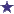# IV Question on Concentration of Solution of Nipride 50 mg

by Sherri
(Raleigh, NC)Nitroprusside is Indicated for Quickly Reducing Blood Pressure in Hypertensive Crises

A 60 yr old woman has hypertension.

Her weight is 165 lbs and her doctor prescribed Nipride 3mcg/kg/min IV.

Nipride 50 mg is added to a 250 ml solution of D5W.

What would be the concentration of Nipride in this solution?

A. 120 mcg/ml
B. 150 mcg/ml
C. 200 mcg/ml
D. 250 mcg/ml.

My book tells me that C. 200 mcg/ml is the answer but how do they get this?

Can someone walk me through this?

### Comments for IV Question on Concentration of Solution of Nipride 50 mg

Average RatingRatingConcentration of Nipride in this Solution is 200mcg/ml by: David First of all, there are 1000 micrograms in 1 milligram. So, the answer is C.) 200mcg/ml. How do we get this? According to the medication insert, Nipride 50mg (Nitropress, Nitroprusside Sodium) is reconstituted with 2-3 ml of sterile water for injection (SWI) (in this case, we will assume that we added 2 ml of SWI.) The reconstituted solution (after adding the SWI) is 25mg/ml (we get this by taking the strength, 50mg and dividing it by the amount of diluent added, 2 mg (the SWI.) 50mg/2ml simplifies to 25mg/1ml.) So, we go back to the beginning of this answer. There are 1000 micrograms in 1 milligram. So we multiply 50mg/2ml by 1000 to get micrograms. 50 mg times 1000 equals 50,000 micrograms. Remember, though, that we have to add this to the 250mg of D5W (which is Dextrose 5% in water). So adding it to the D5W is diluting it. So we divide the 50,000 micrograms by 250ml that it is being added to. 50,000 micrograms divided by 250 (D5W) is 200. So the answer is: The concentration of Nipride in this Solution is 200mcg/ml.